Sunday, April 3, 2011

Quantized Redshifts. X. Testing Our "Designer Universe"

In the last section, I demonstrated, by a simple exercise, how scientists can make model datasets to test their analysis methods.  Of course, once we build these test datasets, we should always conduct some tests on the results to make sure they exhibit the properties we seek for our testing.  That is the purpose of this section.

Consider yourself at the center of a uniform distribution of galaxies (homogeneous), all around you, covering the entire sky (isotropic).  We'll assume they have a constant mean density (number of galaxies per cubic megaparsec) out to the edge of the distribution (homogeneous).  If we have a spherical sampling of galaxies, with a uniform number density, n (galaxies per unit volume), it is easy to compute the number of galaxies per bin, dN, that must be in a thin spherical shell at distance, r, and thickness, dr.

dN = n 4 \pi r^2 dr

This is just the area of the sphere, multiplied by the thickness (to give a volume) all multiplied by the density.

We can compute this function for our array of redshift values using the histogram function.  First we create an array of nbins bins in redshift space (zbinning) out to a maximum value of z, maxz.

zbinning=np.arange(0.0,maxz,maxz/nbins)
distribution=np.histogram(redshift,zbinning)

I plot the result for one of the model runs below with maxz=0.512 and nbins=512.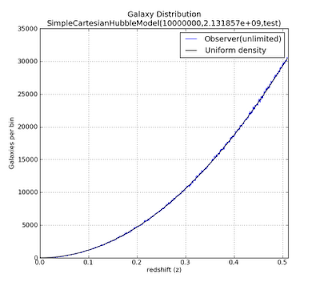Figure 1: Click to enlarge

The blue, slightly irregular, line represents the number of galaxies in each bin of redshift values.  The irregularities are due to the statistical variation in the model.  The black line is an analytic curve of the density distribution.  We see that the two curves have excellent agreement, with the blue curve exhibiting slight statistical fluctuations around the expected count given by the black curve.

This is the kind of distribution of galaxies with redshift, z, that we would expect to observe if we were in a VERY large, VERY uniform universe, and our telescopes have infinite sensitivity, i.e. we see all galaxies no matter how faint.  This is a very unrealistic assumption, and we we will deal with making it more realistic soon.

As an additional test, we can use our distribution counts to compute the density, n, of each radial shell,

n = dN / ( 4 \pi r^2 dr)

We plot the results below.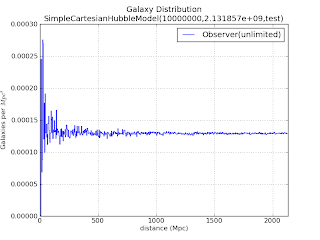Figure 2: click to enlarge

We see that at large distances, the value of n exhibits small fluctuations around the mean density of the input model.  That mean density is computed by dividing the total number of galaxies by the total volume of our model cosmos.  As we sample distances closer to the observer, the fluctuations get larger and larger.  This is not unexpected.  At smaller radii, the number of galaxies in the bin gets smaller and this this makes the statistical error in each bin is larger since it can only have an integer number of galaxies in a bin (no fractional galaxies).   Our input galaxy density is actually less than 1 galaxy per Mpc^3, so when we sample some bins near the center, the probability of having bins with much less (or more) than the mean number of galaxies can show large fluctuations.  After all, at these bin sizes, we can only have integer numbers of galaxies in the bins, either zero or one, but rarely more.

Again, the model behaves as we expect, consistent with our input assumptions.

Making Our Designer Universe More Realistic

But in reality, we do not have telescopes of infinite sensitivity.  To simulate the effect of more realistic telescopes, we will assume the telescopes can only see galaxies brighter than a given limiting apparent magnitude, limitingMagnitude.  For this experiment, we'll choose a limiting magnitude of 18 and 20.  We can plot the distribution of our galaxies in distance vs. apparent magnitude.

First we find the array indices of galaxies in our model catalog that are less than  limitingMagnitude:
magLimitIndices=np.nonzero(apparentMagnitude < limitingMagnitude)

With this array of indices, we can then select out the galaxies from our source arrays, distance, apparentMagnitude, making new arrays, of the galaxies that fall in our magnitude limits.

distances = np.take(distance,magLimitIndices)

apparentMagnitudes = np.take(apparentMagnitude,magLimitIndices)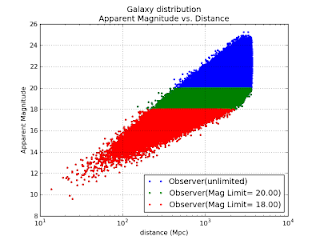Figure 3: click to enlarge

In this plot, the red markers represent all galaxies brighter than magnitude 18.0.  The green band reveals galaxies fainter than magnitude 18 but brighter than magnitude 20, and blue are the remaining galaxies in our catalog.  Note that the distance is a logarithmic scale.  Apparent magnitude is a logarithmic rescaling of energy flux.

At small distances, we count very few galaxies.  This is why our mean density fluctuated so wildly in the bins for near-Earth distances.  Your sampling either caught some galaxies, or it didn't.  The further out we go, we see more galaxies along the vertical line of a fixed distance, up until the point our survey starts missing galaxies because they are too faint.  At this point, the number of galaxies begins to decline until we reach a distance so great that no galaxy is bright enough to be detected.

How that would look in a histogram?

We can compute the mean density again, as above, but this time plotting the result for each different limiting magnitude.  We plot this result below.

distribution=np.histogram(distances,rbinning)
# convert to galaxy density/Mpc^3
density=distribution*1e18/(rbinning[:-1]*rbinning[:-1])/(boundary/nbins)/(4.0*np.pi)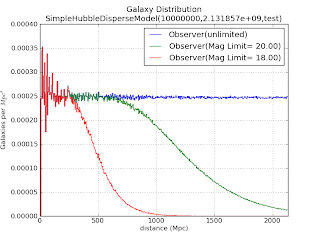Figure 4: click to enlarge

As before, we have large fluctuations in mean density near the Earth, which decreases as the distance from the Earth increases.  But now we have the additional effect of our limiting magnitude of detection.  At some distance, the measured density begins to decrease, as our number of galaxies measured at that distance begins to decrease.  The red, green, and blue curves in Figure 4 represent the corresponding model color in Figure 3.

Food for Thought:  In this exercise, I've examined the case of a survey limited by telescope sensitivity.  Suppose we had telescopes that had a much higher magnitude limit, so our survey probed so deep into the cosmos that we saw fewer galaxies because we were sampling a time when galaxies were just forming?

Now we need to sample this dataset constructed in 3-dimensions, into a radially-sampled dataset as done in the first figure of this article.  We also compute this histogram for our experimental magnitude-limited surveys.

For each of the subsamples above, we can again compute the histogram in redshift-space.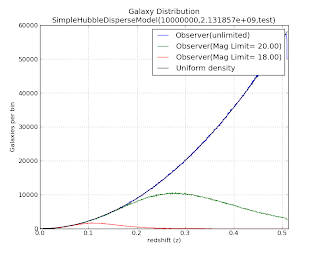Figure 5: click to enlarge

The black curve is the radial galaxy count profile one expects for a uniform density.  The green curve corresponds to the distribution with a limiting magnitude of 20, and the red curve corresponds to a distribution with a limiting magnitude of 18.

Let's zoom in on the red curve, expanding the vertical scale.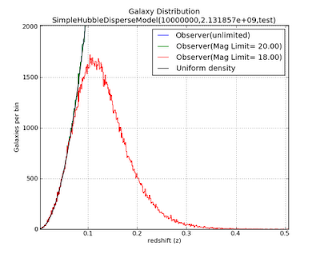Figure 6: click to enlarge

If you've examined a data from a number of large-scale sky surveys, the profile of the red curve might look a bit familiar.

Next (but probably NOT as soon as next weekend): What Can We Learn from this Model and Questions for Further Exploration